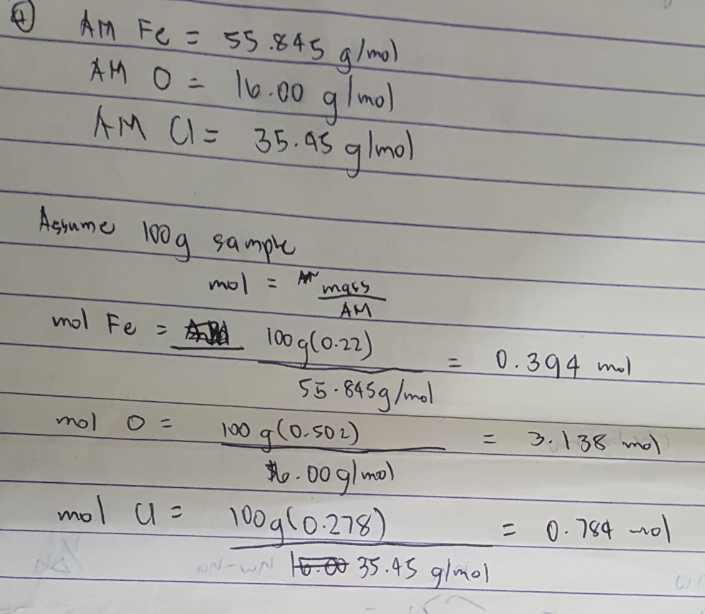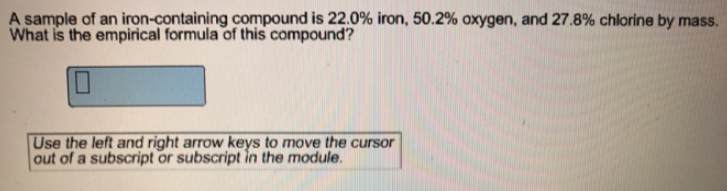Problem: A sample of an iron-containing compound is 22.0% iron, 50.2% oxygen, and 27.8% chlorine by mass. What is the empirical formula of this compound?

FREE Expert Solution
100% (196 ratings)
FREE Expert Solution100% (196 ratings)
Problem Details

A sample of an iron-containing compound is 22.0% iron, 50.2% oxygen, and 27.8% chlorine by mass. What is the empirical formula of this compound?